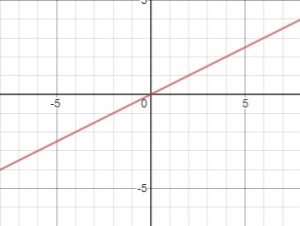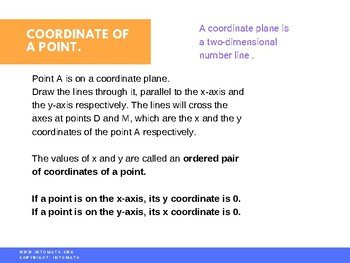CA$2.00 In this lesson you will learn about a coordinate plane (Cartesian Plane). You will learn how to plot a point and record its coordinates – an ordered pair (x, y). You will discover how to determine the relationship between two quantities. One of those quantities is dependent on another like distance and time or like the circumference of a circle and its diameter. You will also learn how to graph the relationship. You will learn about the rate of change (slope of a line) and how to calculate it using the coordinates of the two points. When the relationship is linear, the rate of change is constant.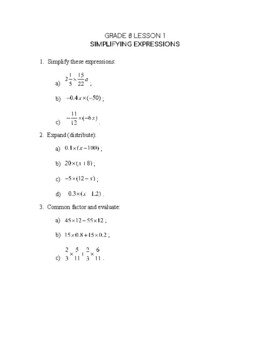Grade 8 Workbook (18 pages with answers) CA$4.00

You will see how the slope of a line (linear relationship) depends on the change in the x and y coordinates.

What are the coordinates of the given point?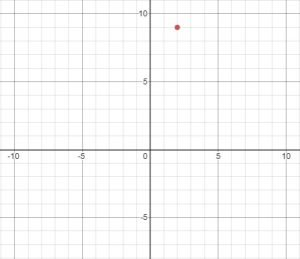What are the coordinates of the given point?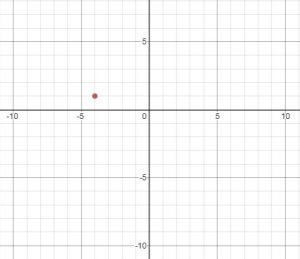What is the slope of this line?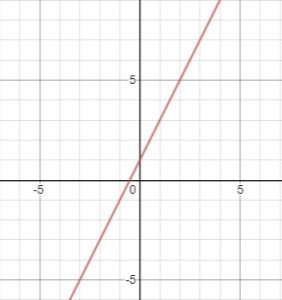What is the slope of this line?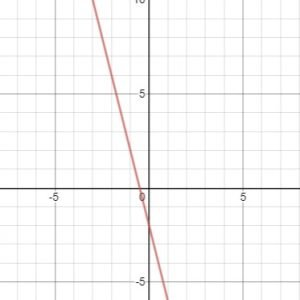What is the slope of this line?